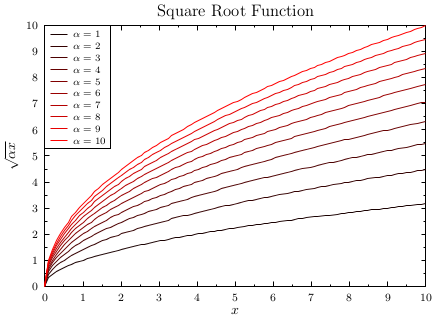GLE Example: sqroot.gle[PDF file]

size 11 8

set texlabels 1
begin graph
scale auto
title "Square Root Function"
xtitle "$x$"
ytitle "$\sqrt{\alpha x}$"
key pos tl hei 0.25
for alpha = 1 to 10
let d[alpha] = sqrt(alpha*x) from 0 to 10
d[alpha] line color rgb(alpha/10,0,0) key "$\alpha = "+num$(alpha)+"\$"
next alpha
end graph